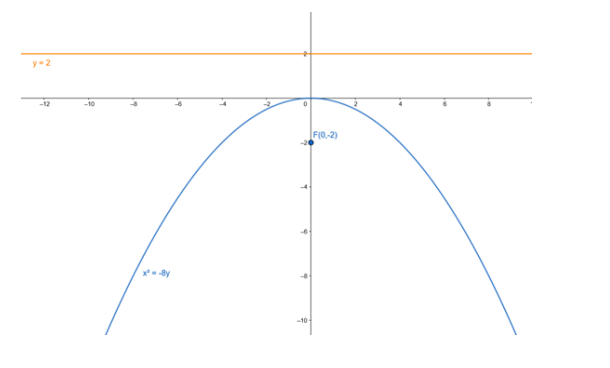# Find the coordinates of the focus and the vertex, the equations of the`
Question:

Find the coordinates of the focus and the vertex, the equations of the directrix and the axis, and length of the latus rectum of the parabola :

$x^{2}=-8 y$

Solution:

Given equation : $x^{2}=-8 y$

Comparing given equation with parabola having equation,

$x^{2}=-4 a y$

$4 a=8$

$\cdot a=2$

Focus: $F(0,-a)=F(0,-2)$

Vertex : $A(0,0)=A(0,0)$

Equation of the directrix : $y-a=0$

- $y-2=0$

- $y=2$

Lenth of latusrectum : $4 a=8$